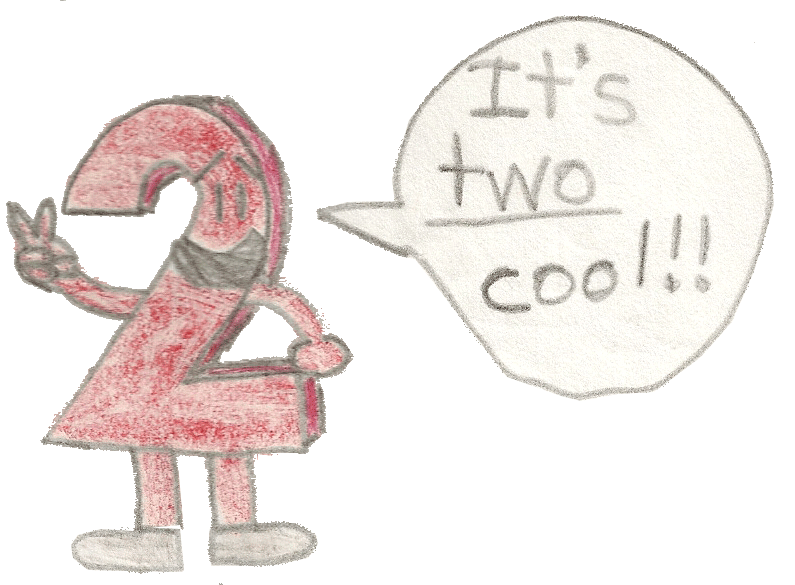# Super Summation 6## Examples:2^0 + 2^1 + 2^2 + 2^3 + 2^4 + 2^5 = 1 + 2 + 4 + 8 + 16 + 32 = 2^6 - 1 = 64 - 1 = 63The caret (^) is the symbol of exponentiation. Raising a non-zero number to the power of zero(0) always gives you 1.2^0 + 2^1 + 2^2 + 2^3 + 2^4 + 2^5 + 2^6 + 2^7 + 2^8 + 2^9 = 1 + 2 + 4 + 8 + 16 + 32 + 64 + 128 + 256 + 512 = 2^10 - 1 = 1,024 - 1 = 1,0232^0 + 2^1 + 2^2 + 2^3 + 2^4 + ... + 2^23 = 1 + 2 + 4 + 8 + 16 + ... + 8,388,608 = 2^24 - 1 = 16,777,216 - 1 = 16,777,215

The triple period was used to save time, of course, from printing all those powers of 2!